## LetsPlayMaths.Com

WELCOME TO THE WORLD OF MATHEMATICS

# Class 3 Time

Recap of Class-II

Reading Time for Exact to 5 Minutes

Reading Time to the Nearest Minute

Use of AM and PM

Conversion of Time

Calendar

Time Test

Time Worksheet

Answer Sheet

## Recap of Class-2

In our day to day life, we use watch/clock to see the time. Figure below shows the face of a clock.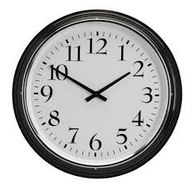We can see there are 12 numbers starting from 1 to 12. We can see there are 5 short divisions between any two consecutive numbers. So, in total we have 60 short divisions. Each short division corresponds to one minute. Every clock has two hands as shown in above figure. The short hand is known has hour hand and the long hand is known as minute hand. The minute hand takes 1 hour to complete one complete rotation. In other word, we can say that minute hand takes 60 minutes to complete one rotation.

So, 60 minutes = 1 hour

Hour hand takes 12 hours to complete one full rotation. Since there are 24 hours in a day, the hour hand completes 2 rotations in a day.

There are some clocks which have additional hand, it is called seconds hand. It completes one round in 1 minute.

So, 60 seconds = 1 minutes

Here we will discuss on hour and minute hand.

## Reading Time for Exact to 5 Minutes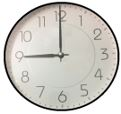In the above figure, the hour hand is at 9 and the minute hand is at 12.
So, the time is 9 o’clock.
It is written as 9:00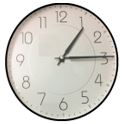In the above figure, the hour hand is between 1 and 2. The minute hand is at 3. The time is 15 minutes past 1 or one-fifteen. We write it as 1:15.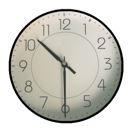In the above figure, the hour hand is between 10 and 11. The minute hand is at 6. The time is 30 minutes past 10 or half past 10. We write it as 10:30.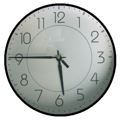In the above figure, the hour hand is between 5 and 6. The minute hand is at 9. The time is 45 minutes past 5 or five forty-five. It is written as 5:45, also called as quarter to 6.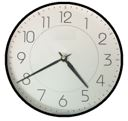In the above figure, the hour hand is between 4 and 5. The minute hand is at 8. The time is 40 minutes past 4. We say it four forty and we write 4.40.

## Reading Time to the Nearest MinuteIn the above figure, the hour hand is between 9 and 10. That means the time is between 9 o’ clock and 10 o’ clock. The minute hand is between 1 and 2, it is 4 small divisions ahead of the number 1. That means, minute hand has covered 5 + 4 or 9 small divisions after 9 o’ clock. So, the time will be 9 minutes past 9.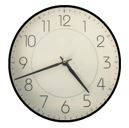In the above figure, the hour hand is between 4 and 5. The minute hand is between 8 and 9 and it is 2 divisions ahead of number 8. That means, the minute hand has covered (8X5) +2 or 42 divisions ahead of the number 4. So,the time is 42 minutes past 4 or four forty-two.

## Use of AM and PM

As we know a day has 24 hours, but only 12 hours are shown on the face of the clock. So, the day is divided into two halves. From 12 o’ clock midnight to 12 o’ clock noon it is considered as first half. These 12 hours are considered as morning hours. So, anytime shown between midnight and noon is written as AM.

From 12 noon to 12 midnight is the second half. These 12 hours are called as evening hours. To show anytime from 12 noon to 12 midnight we write PM.

## Conversion of Time

Till now we knew following things.

1 minutes = 60 seconds

1 hour = 60 minutes

1 day = 24 hours

Example 1. Convert 5 hours into minutes

Solution.
1 hour = 60 minutes
5 hours = 60 X 5 = 300 minutes

Example 2. Convert 6 hours 25 minutes into minutes

Solution.
6 hours = 60 X 6 = 360 minutes
6 hours 25 minutes = 360 + 25 = 385 minutes

Example 3. Convert 95 minutes into hours and minutes

Solution.
95 minutes = 60 minutes + 35 minutes
= 1 hour 35 minutes

## Calendar

In previous classes we learnt that there are 7days in a week. The days of the week are given below.

1. Monday
2. Tuesday
3. Wednesday
4. Thursday
5. Friday
6. Saturday
7. Sunday

There are 12 months in a year and they are mentioned below.

1. January - 31 days
2. February - 28 days or 29 days (Leap Year)
3. March - 31 Days
4. April - 30 Days
5. May - 31 Days
6. June - 30 Days
7. July - 31 Days
8. August - 31 Days
9. September - 30 Days
10. October - 31 Days
11. November - 30 Days
12. December - 31 Days

There are 365 days in a year, but in a leap year there are 366 days.Leap year comes once in 4 years.
In a leap year February month has 29 days.

Time Test - 1

Time Test - 2

Time Test - 3

## Class-3 Time Worksheet

Time Worksheet - 1

## Answer Sheet

Time-AnswerDownload the pdf

Copyright © 2021 LetsPlayMaths.com. All Rights Reserved.